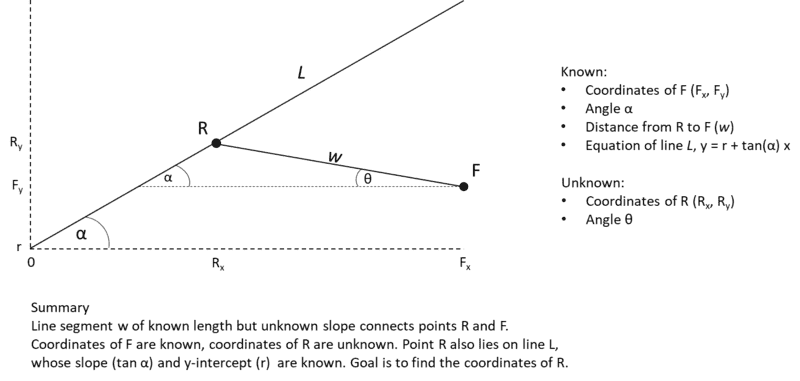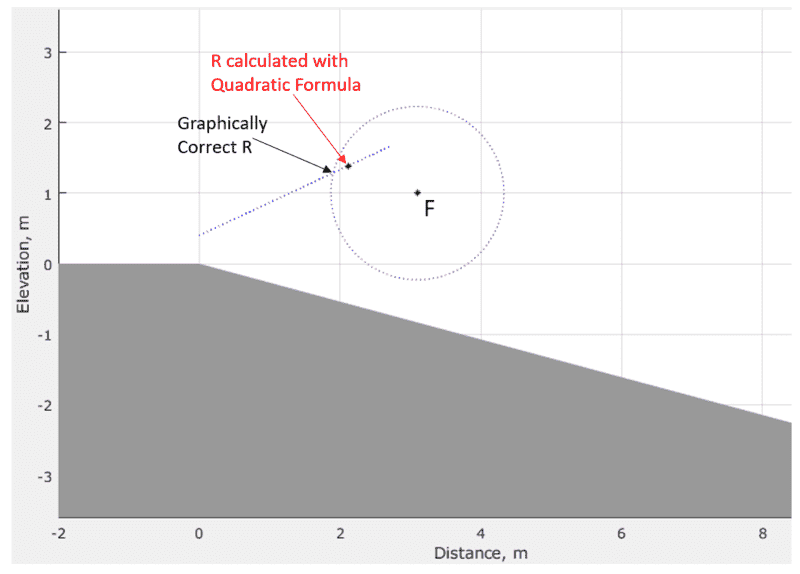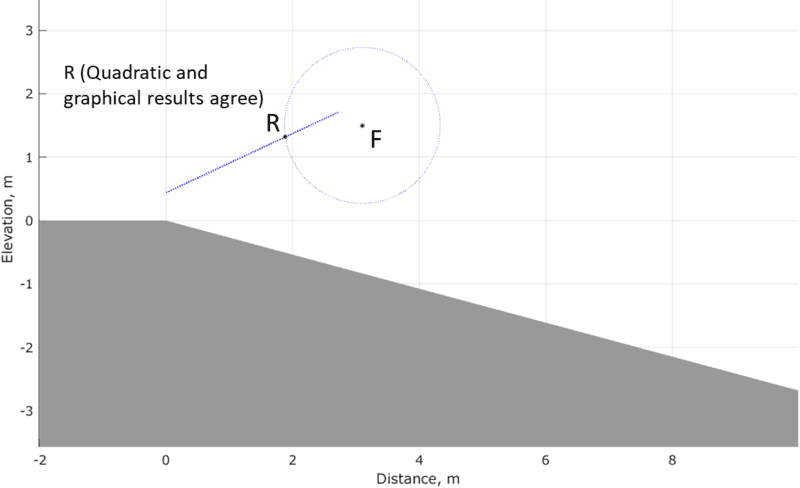# Geometry/Trig Problem -- Well Constrained but Difficult

• I
steveZ
The image below should explain the problem and the constraints. Basically, I know the location of one point (F) in 2-D space (Cartesian coordinates). A line segment (w) of known length connects this point to another point (R). The coordinates of R are unknown; however, it is known to lie on a line (L), the slope and y-intercept of which are known (tanα and r). My intuition is that this should be solvable and not all that complicated. The slope of segment w is unknown, but there should only be one angle that allows a segment of that length to exactly reach line L. But I cannot figure it out so I am appealing to the community.#### Attachments

Mentor
But I cannot figure it out so I am appealing to the community.
Can you show us what you've tried so far? Is this for schoolwork, or a personal project?

Also, are you familiar with how to convert between rectangular and polar coordinates, and vector representations in each set of coordinates?

Mentor
A line segment (w) of known length connects this point to another point (R). The coordinates of R are unknown; however, it is known to lie on a line (L), the slope and y-intercept of which are known (tanα and r). My intuition is that this should be solvable and not all that complicated. The slope of segment w is unknown, but there should only be one angle that allows a segment of that length to exactly reach line L.
The equation of the line is ##y = \tan(\alpha)x + y_0##, where ##y_0## is the known y-intercept. A circle of radius w is ##(x - F_x)^2 + (y - F_y)^2 = w^2## will intersect the line at two points, one of which is the point you seek. One of these line segments will have a slope that is an acute angle, and the other will have an angle that is greater than 90°.

•Lnewqban and steveZ
Gold Member
2022 Award
The equation of the line is ##y = \tan(\alpha)x + y_0##, where ##y_0## is the known y-intercept. A circle of radius w is ##(x - F_x)^2 + (y - F_y)^2 = w^2## will intersect the line at two points, one of which is the point you seek. One of these line segments will have a slope that is an acute angle, and the other will have an angle that is greater than 90°.
Mark, I thought we were supposed to wait for an OP to show some work before we start giving them hints. Have the rules changed?

Mentor
No, the rules haven't changed. It looked to me like he had organized the information he had and what was unknown. I was just pointing him in a direction to reach the answer.

•steveZ, jedishrfu and phinds
steveZ
Can you show us what you've tried so far? Is this for schoolwork, or a personal project?

Also, are you familiar with how to convert between rectangular and polar coordinates, and vector representations in each set of coordinates?
It's for a personal project. I have tried many things, including setting equations for the two lines equal to each other, substitutions to reduce the number of unknowns, etc. The closest I've come was to get a horrendously complicated quadratic equation with Rx^2 and Rx.
I am not comfortable with polar coordinates or transformations from rectangular to polar. Does this look like the approach that is needed?

Mentor
Does this look like the approach that is needed?
Did you consider Mark's hinted method?

steveZ
Mark, I thought we were supposed to wait for an OP to show some work before we start giving them hints. Have the rules changed?
I have filled many sheets of paper with attempts to solve using algebra and trigonometry but did not want to bore you with the many blind alleys I have navigated. This is not a "homework" problem. What is an OP?

steveZ
The equation of the line is ##y = \tan(\alpha)x + y_0##, where ##y_0## is the known y-intercept. A circle of radius w is ##(x - F_x)^2 + (y - F_y)^2 = w^2## will intersect the line at two points, one of which is the point you seek. One of these line segments will have a slope that is an acute angle, and the other will have an angle that is greater than 90°.
Thank you very much, Mark. This looks promising and I will follow up on it.

steveZ
Did you consider Mark's hinted method?
I absolutely will. I replied to your post before I saw his.

Mentor
What is an OP?
Original post or original poster

steveZ
Of course, thanks. I like the idea of thinking of a circle with radius w with origin at point F that includes point R. In fact, the physical meaning of this problem came from realizing that, if you do it backwards and start with known R, there is a circle of radius w comprising possible points (including F) determined by the angle of the connecting segment w. So I should have thought of that.

So now, as I see it, I have two equations for two unknowns. The circle equation has two unknowns, Rx and Ry, but the equation of line L gives me Ry as a function of Rx, so I can substitute. So I have:
w2 = (Rx - Fx)2 + (y0 + tanαRx - Fy)2
I recognize this as an equation I managed to get to yesterday by a longer route. I pursued it then but got a bit lost in the number of terms that result when you square a binomial and a trinomial (10 terms altogether when you multiply it out, including LHS and RHS).

This is messier than I had hoped, since I want to code this in Matlab, and it is just a small piece of a much larger problem. By rearranging, and separating out the known quantities, I can get this into the quadratic form:
0 = ax2 + bx + c
Then I can use the quadratic formula to get the two possible values. However, the constant c comprises 5 terms, b has 3 and constant a comprises 2 terms, so it is a bit tedious. Is this what you had in mind with the great hint you gave me earlier? Or am I missing a way to simplify the solution? Thanks again.

Last edited:
Mentor
w2 = (Rx - Fx)2 + (y0 + tanαRx - Fy)2
I recognize this as an equation I managed to get to yesterday by a longer route. I pursued it then but got a bit lost in the number of terms that result when you square a binomial and a trinomial (10 terms altogether when you multiply it out, including LHS and RHS).
But w, Fx, Fy, and ##\alpha## are known, so you could conceivably substitute in the known values for these constants (including ##\tan(\alpha)##). Or not...
This is messier than I had hoped, since I want to code this in Matlab, and it is just a small piece of a much larger problem. By rearranging, and separating out the known quantities, I can get this into the quadratic form:
0 = ax2 + bx + c
Then I can use the quadratic formula to get the two possible values. However, the constant c comprises 5 terms, b has 3 and constant a comprises 2 terms, so it is a bit tedious. Is this what you had in mind with the great hint you gave me earlier?
Yes, you will end up with a quadratic equation in Rx, and then use the quadratic formula to solve for Rx.

•steveZ
Homework Helper
Gold Member
Last edited:
•steveZ
valenumr
Given alpha, you can make a right triangle, which gives you theta. Since you know the hypotenuse length, you should be able to calculate all parameters of the triangle.

steveZ
Given alpha, you can make a right triangle, which gives you theta. Since you know the hypotenuse length, you should be able to calculate all parameters of the triangle.
I wish that were so, but if you look at the diagram again, I think you will see that it is not so easy.

•valenumr
valenumr
Given alpha, you can make a right triangle, which gives you theta. Since you know the hypotenuse length, you should be able to calculate all parameters of the triangle.
Oh silly me... It looks like your problem only specifies one side length and the opposite angle. I'd be interested to see if this is solvable with the other info provided. I got thrown off a bit by the diagram showing the bottom as horizontal.

valenumr
I wish that were so, but if you look at the diagram again, I think you will see that it is not so easy.
What I'm seeing, one could imagine moving the corner of the triangle to the origin, and reducing the problem to solving a triangle with only one known side length and one known angle (which is not solvable). The offset along R seems irrelevant in the grand scheme of things.

valenumr
Ok, it makes sense now... You can move the point R pretty much anywhere along the line L and still keep length w constant because theta is unspecified. There is no unique solution to this problem.

valenumr
Ok, it makes sense now... You can move the point R pretty much anywhere along the line L and still keep length w constant because theta is unspecified. There is no unique solution to this problem.
Darnit.. the point f is specified... So it should be solvable.

valenumr
The solution is now in focus... Just think of the bottom left of the triangle as the origin and compute the triangle parameters in that coordinate system. For example, you know the base length is Fx - Rx. That gives you three parameters. It still seems messy.

Gold Member
2022 Award
I can't see what everyone is going on about. The solution is conceptually trivial (@Mark44 gave the whole solution in post #3 and then expounded on it in post #13), it's just algebraically a mess.

•Lnewqban
steveZ
I can't see what everyone is going on about. The solution is conceptually trivial (@Mark44 gave the whole solution in post #3 and then expounded on it in post #13), it's just algebraically a mess.
You are exactly right. I have done the solution but, because of the messy algebra, it is easy to make mistakes. I have written code to plot my results and also the circle and line whose intersection we are trying to find. You can see in the image below that there is a small error that I am trying to nail down.•phinds
Mentor
Given alpha, you can make a right triangle, which gives you theta. Since you know the hypotenuse length, you should be able to calculate all parameters of the triangle.
I don't see how this is a viable approach.
You can move the point R pretty much anywhere along the line L and still keep length w constant because theta is unspecified.
No, you can't move R along the line. Doing so would affect the length of w.
I have written code to plot my results and also the circle and line whose intersection we are trying to find. You can see in the image below that there is a small error that I am trying to nail down.
That error looks pretty significant to me, maybe .3 meter or so. I haven't done the calculations, but I would guess that the incorrect results stem from calculation errors.

Mentor

Mentor
After a brief Mentor discussion, the thread us re-opened...

steveZ
I don't see how this is a viable approach.

No, you can't move R along the line. Doing so would affect the length of w.

That error looks pretty significant to me, maybe .3 meter or so. I haven't done the calculations, but I would guess that the incorrect results stem from calculation errors.
It was a mistake I made when doing the algebra. A factor of two on one of the terms that went into the quadratic. I caught it on my third try solving for R. Here is the latest result, which seems correct. Thanks again for your help.#### Attachments

•Lnewqban
Mentor
It was a mistake I made when doing the algebra.
Easy to do with such a complicated equation to solve, but good job on your correction.